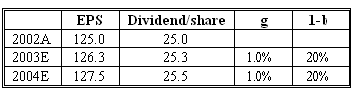### Seeing is believing!

Before you order, simply sign up for a free user account and in seconds you'll be experiencing the best in CFA exam preparation.

##### Subject 3. The Gordon Growth Model
It's impossible to forecast all future dividends infinitely. If we assume the future dividend stream will grow at a constant rate g for an infinite period, r is greater than g, and D1 is the dividend to be received at the end of period 1, then:The crucial relationship that determines the value of the stock is the spread between the required rate of return (r) and the expected growth rate of dividends (g). Anything that causes a decline in the spread will cause an increase in the computed value, whereas any increase in the spread will decrease the computed value.

Notice that the expression has next year's dividend, D1, in the numerator. If the payout rate and ROE are constant value, dividends and earnings grow at the same rate g. Dividends may fall at a constant rate indefinitely extending in the future, for example if a company's resources are depleting. In this case the formula will still be valid and g will be negative.

This model was developed by Myron J Gordon and is called the Gordon Growth Model (GGM).

The formula does not work for companies with dividend growth in excess of the required rate of return. For such "growth companies" analysts employ two-stage or three-stage models. Usually companies eligible for evaluation using the GGM grow at a rate close to the expected long-term growth of gross domestic product (which consists of real growth and expected inflation).

The process of estimating the inputs to be used in the model:

• Estimate the required rate of return (r):

• Estimate the real risk-free rate.
• Estimate the expected rate of inflation.
• Calculate the nominal risk-free rate: (1 + real risk-free rate) x ( 1 + expected rate of inflation) - 1.
• Estimate the risk premium of the stock.
• Calculate the required rate of return on the stock: nominal risk-free rate + risk premium.

• Estimate the dividend growth rate (g):

• Estimate the firm's retention ratio.
• Estimate the firm's expected return on equity (ROE).
• Calculate the dividend growth rate: Retention rate (b) x Return on equity (ROE)

Expected rate of return implied in the Gordon growth model can be calculated by assuming that value equals market price and solving the general GGM equation for r:

r = D1/P0 + g (1), or alternatively,

r = D1/P0 + (P1 - P0)/P0 (2)

Equation (1) expresses the expected rate of return as the sum of the expected dividend yield and the dividend growth rate. Realizing that the company price grows at the same rate g (provided that markets are efficient), we can also use equation (2), which shows that the expected rate of return is a sum of the dividend yield and the expected capital gains yield.

Example

An analyst is estimating company X's future earnings and dividends. The company is currently trading at \$320. He believes that the dividend growth will be 1% from 2003 and thereafter.The analyst wants to compare the expected rate of return implied in the Gordon growth model with the required rate of return of 10%.

We can estimate the expected rate of return using formula (1): r = D2003 / P2002 + g = 25.3/320 + 0.01 = 0.089, or 8.9%.

This rate is lower than the required rate of return, 10%, therefore the company is not an attractive investment opportunity.

Calculating the value of fixed-rate perpetual preferred stock.

Fixed-rate perpetual preferred stock is a senior claim on a company's assets comparing to its current stock. It entitles stockholders for a level stream of dividends in perpetuity. Payments of fixed-rate perpetual preferred dividends are made only after the firm pays its bond interest. Thus,

Preferred Stock Value = Dividend / r

r is sometimes called capitalization rate.

The Gordon growth model is most suitable when:

• The company has established record of dividend payouts.
• Dividend payments and earnings are growing at a stable rate.

Strengths:

• GGM very clearly and simplistically defines the relationships among value and growth, required rate of return and payout ratio.
• It allows us easily perform valuation of stable-growth, dividend-paying companies.
• It can be used for valuation of broad-based equity market indexes.
• It is useful for estimation of terminal value in multiple-stage models.

Limitations:

• GGM is highly sensitive to growth rate and required rate of return inputs. Very small variations in estimation of these inputs can lead to significant different values estimates.
• It can not be used to value non-dividend paying stocks.
• It can not be used to value companies with unstable growth rates.

User Comment
MasterD Additional limitation though not "textbook": Does not work if g > r because that would lead to a negative P/E ratio.
bmeisner The first example shows how stupid the DDM/GGM is, the company is trading at 3x earnings and the model tells you it is overvalued. Clearly if someone bought control of the whole company below 10x earnings, the required rate of return would be fulfilled.
Querdenker Very true.
rhardin Actually, that is textbook. It's stated in the notes and the CFA required reading as a problem associated with the GGM.
merc5559 @bmeisner it is very disappointing you would say such rude things about Myron's model. He had feelings too you know?
thebkr777 For the sake of argument only, not all earnings are equal
davidt876 where u been MasterD? use multiple stage growth model if g>r for a certain period.

the perpetuity formula doesn't work for g>r, because g can't be larger than r forever. so it's less a limitation, and more a logical rule built into the formula